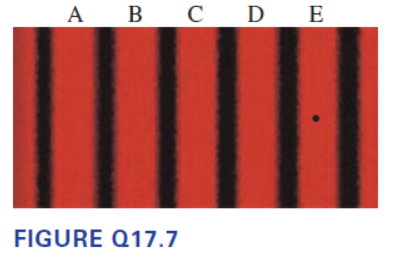# Problem: Figure Q17.7 shows the viewing screen in a double-slit experiment with monochromatic light.Fringe C is the central maximum. What will happen to the fringe if the wavelength of the light is decreased?

###### FREE Expert Solution

Constructive interference is expressed as:

Where d is the separation between the two slits, m is the order, and λ is the wavelength.

For small angles measured in radians, θ ≃ sin θ ≃ tan θ ≃ y/L

92% (476 ratings)###### Problem Details

Figure Q17.7 shows the viewing screen in a double-slit experiment with monochromatic light.

Fringe C is the central maximum.

What will happen to the fringe if the wavelength of the light is decreased?